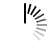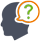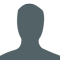Diwali Bonanza | Get 30-Days Free Fun-Filled Learning | Subscribe Now1800-833-6464 Buy Activation Why Tutorix Features Login

#DoubtTushar

In figure below, $AB\ ∥\ QR$, find the length of $PB$.Solutions :Akhilesh

Given:

In the given figure $AB\ ∠¥\ QR$.

To do:

We have to find the length of $PB$.

Solution:

$AB = 3\ cm, QR = 9\ cm$ and $PR = 6\ cm$

In $\vartriangle PAB$ and $\vartriangle PQR$,

$\angle P = \angle P$  (Common)

$\angle PAB = \angle PQR$ ($AB||QR$, Corresponding angles)

Therefore,

$\vartriangle PAB ∠¼ \vartriangle PQR$   (By AA similarity)

Hence,

$\frac{AB}{QR} = \frac{PB}{PR}$ (Corresponding parts of similar triangles are proportional)

$\frac{3}{9} = \frac{PB}{6}$

$PB = \frac{6}{3}$

$PB = 2\ cm$

The value of $PB$ is $2\ cm$.

0

﻿